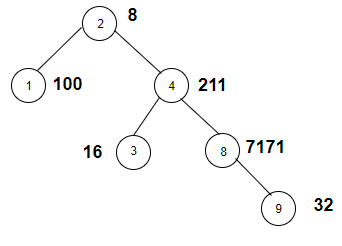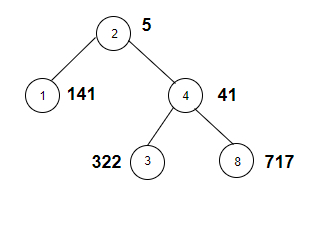# Count the nodes in the given tree whose weight is a power of two in C++

Given a binary tree with weights of its nodes. The goal is to find the number of nodes that have weights such that the number is power of two. If weight is 32 then it is 25 so this node will be counted.

For Example

## Input

The tree which will be created after inputting the values is given below −## Output

Count the nodes in the given tree whose weight is a power of two are: 3

## Explanation

we are given with the tree node and the weights associated with each
node. Now we calculate the power of each and every weight and check whether it can
be expressed as power of 2 or not.

NodeWeightWeight^2Power of 2
282*2*2no
110010*2yes
4211Prime numberno
3164^2yes
81717Not possibleno
9322*2*2*2*2yes

## Input

The tree which will be created after inputting the values is given below −## Output

Count the nodes in the given tree whose weight is a power of two are: 3

## Explanation

we are given with the tree node and the weights associated with each
node. Now we calculate the digit sum of each and every weight and check whether it's
odd or not.

NodeWeightWeight ^ 2Power of 2
2164^2yes
1141Not possibleno
441Prime numberno
3648^2yes
8819^2yes

Approach used in the below program is as follows

In this approach we will apply DFS on the graph of the tree to traverse it and check if the weight of nodes is power of 2. Take two vectors Node_Weight(100) and edge_graph for this purpose.

• Initialize Node_Weight[] with the weights of nodes.

• Create a tree using vector edge_graph.

• Take a global variable sum and initialize it with 0.

• Function power_two(int node, int root) takes a node and root node of a tree and returns the count of nodes in the given tree whose weight is power of two.

• Take set = Node_Weight[node];

• If set && (!(set & (set − 1))) returns true then it is power of two (bitwise AND and then negation)

• Increment powers as set has a value which is power of 2.

• Traverse tree in vector edge_graph[node] using for loop.

• Call power_two(it, node) for the next node in the vector.

• At the end of all functions we will have power as the number of nodes with weights having value as power of two.

## Example

Live Demo

#include <bits/stdc++.h>
using namespace std;
vector<int> Node_Weight(100);
vector<int> edge_graph;
int powers = 0;
void power_two(int node, int root){
int set = Node_Weight[node];
if(set && (!(set & (set − 1)))){
powers++;
}
for(int it : edge_graph[node]){
if(it == root){
continue;
}
power_two(it, node);
}
}
int main(){
//weight of the nodes
Node_Weight = 8;
Node_Weight = 100;
Node_Weight = 211;
Node_Weight = 16;
Node_Weight = 7171;
Node_Weight = 32;
//create graph edge
edge_graph.push_back(1);
edge_graph.push_back(4);
edge_graph.push_back(3);
edge_graph.push_back(8);
edge_graph.push_back(9);
power_two(2, 2);
cout<<"Count the nodes in the given tree whose weight is a power of two are: "<<powers;
return 0;
}

## Output

If we run the above code it will generate the following output −

Count the nodes in the given tree whose weight is a power of two are: 3